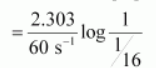# The rate constant for a first order reaction is

Question:

The rate constant for a first order reaction is 60 s−1. How much time will it take to reduce the initial concentration of the reactant to its 1/16th value?

Solution:

It is known that,

$t=\frac{2.303}{k} \log \frac{[\mathrm{R}]_{0}}{[\mathrm{R}]}$$=\frac{2.303}{60 \mathrm{~s}^{-1}} \log 16$

$=4.6 \times 10^{-2} \mathrm{~s}$ (approximately)

Hence, the required time is 4.6 × 10−2 s.